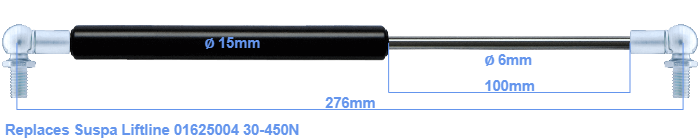# Replacement for Suspa Liftline 01625004 30-450N

\$31.55

Replacement gas spring for the Suspa Liftline 01625004 30-450 Newton. This fits a (possibly already present) ball with a diameter of 10mm. A corresponding ball is included. Brand: Stabilus Industry Line.
 Force Choose an option30 Newton40 Newton50 Newton60 Newton80 Newton100 Newton120 Newton140 Newton150 Newton160 Newton180 Newton200 Newton220 Newton240 Newton250 Newton260 Newton280 Newton300 Newton320 Newton340 Newton350 Newton360 Newton380 Newton400 Newton420 Newton440 Newton450 NewtonClear
This gas spring is also known as 16-1 016 25004, 16-1-131-104-A246-B246.The diameter of the cylinder of this gas spring is 15 millimeters. The diameter of the rod totals 6 mm. The stroke (the retracting part) has a length of 100 mm. In total, the length is 276 millimeter. Attention: this is the length between the centers of the mounting parts. Without the mounting parts this gas spring is 232 mm long (thread to thread). The force of this replacement gas spring is 30-450 Newton. This is not an original gas spring produced by Suspa Liftline, but it can replace a Suspa Liftline gas spring. This is a Stabilus Industry Line gas spring. The force (30-450 N) and dimensions are identical to the original.
Category: